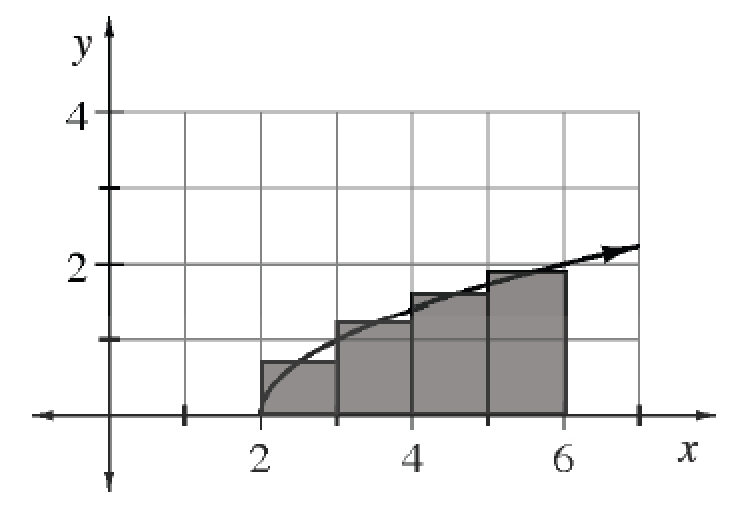### Home > APCALC > Chapter 2 > Lesson 2.1.1 > Problem2-9

2-9.

For $f(x) = \sqrt {x-2}$, he estimation of the area under the curve for $2 \le x \le 6$ is shown at right using four midpoint rectangles. Approximate the area using these rectangles. How reasonable is your result?

$A = \text{(base)(height)}$
$\text{Base}=1$ for all rectangles. Height is determined by the function, evaluated at midpoints.

$\text{The height of the smallest rectangle is }\sqrt{0.5}.$

$\text{The height of the largest rectangle is }\sqrt{3.5}.$